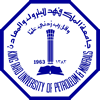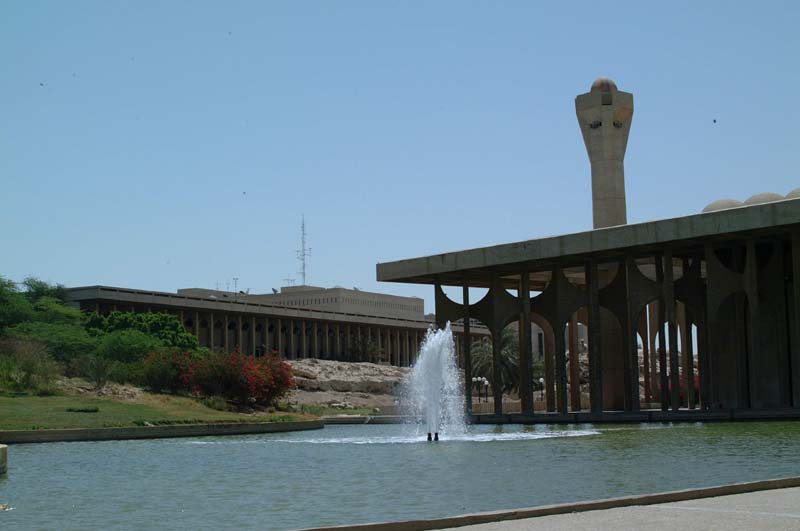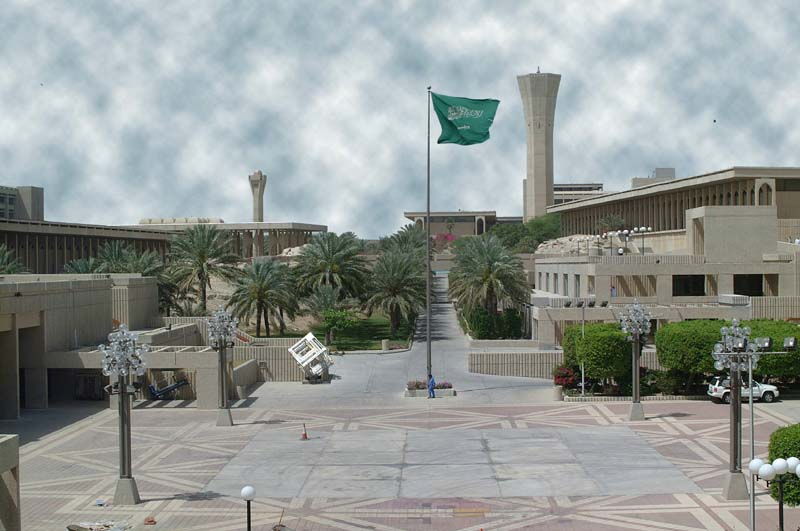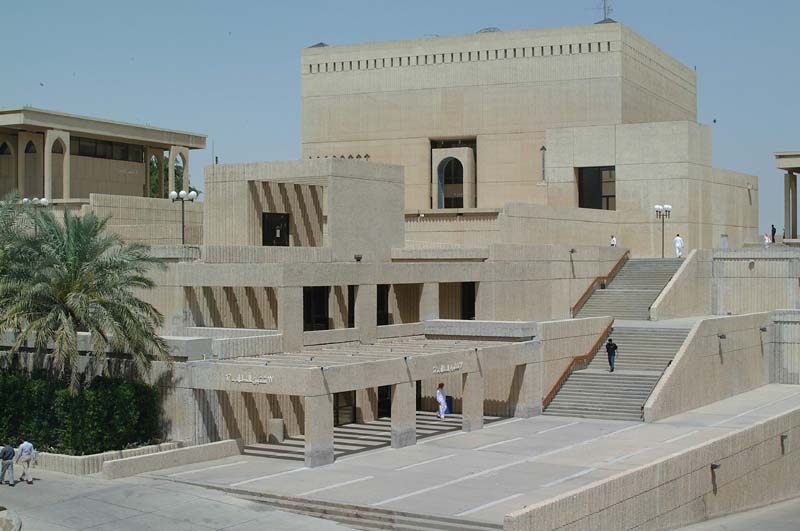﻿ DR. BEG | KFUPM | Teaching

Office:                       5-306
Telephone:        (966) 3 860 2648
Email:     kabir@kfupm.edu.sa

King Fahd University of Petroleum & Minerals
Department of Mathematical Science## Teaching

### Present Courses (072)

Math-102 Calculus II (Syllabus)

### Past Courses

Bangladesh University of Engineering & Technology

• Differential and Integral Calculus with Applications (10 terms)

• Ordinary Differential Equations (4 terms)

• Numerical Analysis (8 terms)

• Complex Variables (2 terms)

• Numerical Analysis (1 term)

King Fahd University of Petroleum & Minerals
Dhahran, Saudi Arabia

• Preparatory Math I and II (22 terms)

• Calculus I (4 terms)

• Calculus II (7 terms)

• Calculus III (1 term)

• Differential Equations (2 terms)

• Differential Equations & Linear Algebra (3 terms)

• Finite Mathematics and Applied Calculus (7 terms)

• Numerical Computing (3 terms)

• Engineering Mathematics (1 term)

### Resources